Next: Energy-Shifts and Decay-Widths Up: Time-Dependent Perturbation Theory Previous: Dyson Series

# Sudden Perturbations

Consider, for example, a constant perturbation that is suddenly switched on at time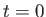: that is,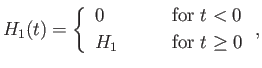(8.61)

where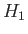is independent of time, but is generally a function of the position, momentum, and spin operators. Suppose that the system is definitely in state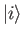at time. According to Equations (8.58) and (8.59) (with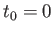),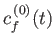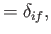(8.62)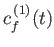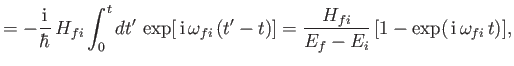(8.63)

giving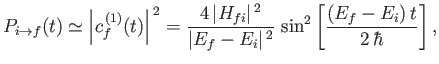(8.64)

for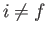. The transition probability between statesand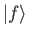can be written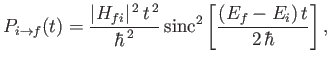(8.65)

where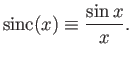(8.66)

The sinc function is highly oscillatory, and decays like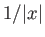at large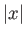. In fact, it is a good approximation to say that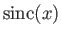is small compared to unity except when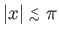. It follows that the transition probability,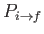, is negligibly small unless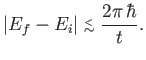(8.67)

Note that, in the limit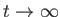, only those transitions that conserve energy (i.e.,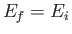) have an appreciable probability of occurrence. At finite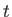, is is possible to have transitions that do not exactly conserve energy, provided that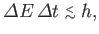(8.68)

where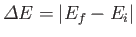is the change in energy of the system associated with the transition, and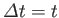is the time elapsed since the perturbation was switched on. This result is a manifestation of the well-known uncertainty relation for energy and time . Incidentally, the energy-time uncertainty relation is fundamentally different from the position-momentum uncertainty relation because (in non-relativistic quantum mechanics) position and momentum are operators, whereas time is merely a parameter.

The probability of a transition that conserves energy (i.e.,) is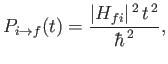(8.69)

where use has been made of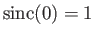. Note that this probability grows quadratically in time. This result is somewhat surprising, because it implies that the probability of a transition occurring in a fixed time interval,to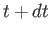, grows linearly with, despite the fact thatis constant for. In practice, there is usually a group of final states, all possessing nearly the same energy as the energy of the initial state,. It is helpful to define the density of states,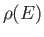, where the number of final states lying in the energy range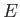to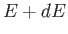is given by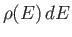. Thus, the probability of a transition from the initial stateto one of the continuum of possible final states is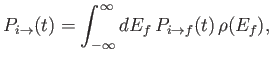(8.70)

giving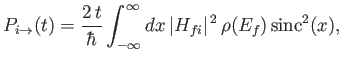(8.71)

where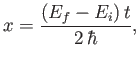(8.72)

and use has been made of Equation (8.68). We know that, in the limit, the functionis only non-zero in an infinitesimally narrow range of final energies centered on. It follows that, in this limit, we can take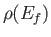and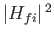out of the integral in the previous formula to obtain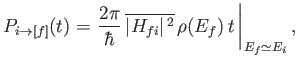(8.73)

where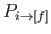denotes the transition probability between the initial state,, and all final states,, that have approximately the same energy as the initial state. Here,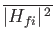is the average ofover all final states with approximately the same energy as the initial state. In deriving the previous formula, we have made use of the result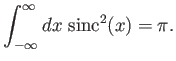(8.74)

Note that the transition probability,, is now proportional to, instead of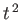.

It is convenient to define the transition rate, which is simply the transition probability per unit time. Thus,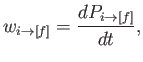(8.75)

which implies that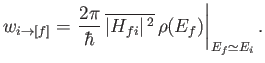(8.76)

This appealingly simple result is known as Fermi's golden rule [29,44]. Note that the transition rate is constant in time (for): that is, the probability of a transition occurring in the time intervaltois independent offor fixed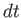. Fermi's golden rule is more usually written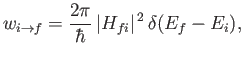(8.77)

where it is understood that this formula must be integrated with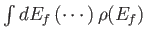in order to obtain the actual transition rate.

Let us now calculate the second-order term in the Dyson series, using the constant perturbation (8.64). From Equation (8.60), we find that (with)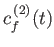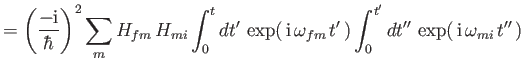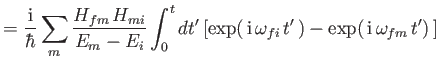(8.78)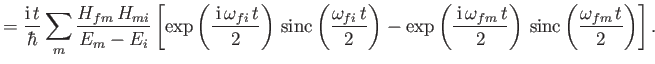Thus,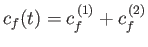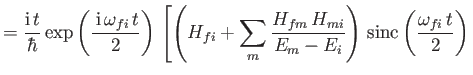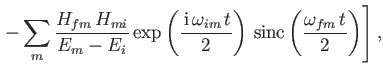(8.79)

where use has been made of Equation (8.66). It follows, by analogy with the previous analysis, that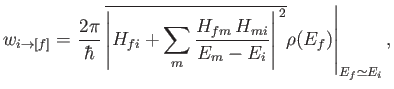(8.80)

where the transition rate is calculated for all final states,, with approximately the same energy as the initial state,, and for intermediate states,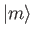whose energies differ from that of the initial state. The fact that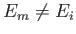causes the final term on the right-hand side of Equation (8.82) to average to zero during the evaluation of the transition probability. (See Exercise 4.)

According to Equation (8.83), a second-order transition takes place in two steps. First, the system makes a non-energy-conserving transition to some intermediate state. Subsequently, the system makes another non-energy-conserving transition to the final state. The net transition from stateto stateconserves energy. The non-energy-conserving transitions are generally termed virtual transitions, whereas the energy conserving first-order transition is termed a real transition. The previous formula clearly breaks down if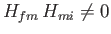when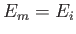. This problem can be avoided by gradually turning on the perturbation: that is,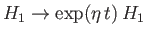(where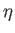is very small). The net result is to change the energy denominator in Equation (8.83) from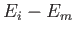to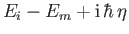.Next: Energy-Shifts and Decay-Widths Up: Time-Dependent Perturbation Theory Previous: Dyson Series
Richard Fitzpatrick 2016-01-22